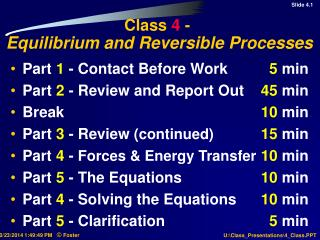DownloadDownload PresentationClass 4 - Equilibrium and Reversible Processes

# Class 4 - Equilibrium and Reversible Processes

Download Presentation## Class 4 - Equilibrium and Reversible Processes

- - - - - - - - - - - - - - - - - - - - - - - - - - - E N D - - - - - - - - - - - - - - - - - - - - - - - - - - -
##### Presentation Transcript

1. Class 4 - Equilibrium and Reversible Processes • Part 1 - Contact Before Work 5 min • Part 2 - Review and Report Out 45 min • Break 10 min • Part 3 - Review (continued)15 min • Part 4 - Forces & Energy Transfer10 min • Part 5 - The Equations10 min • Part 4 - Solving the Equations 10 min • Part 5 - Clarification 5 min

2. Begin With the End in Mind :Class Learning Objectives • Demonstrate Knowledge LoL for • equilibrium(mechanical, thermal, electrical), • an internally reversible process, • a reversible process. • what causes a process to be irreversible, • the concept of ‘immediatesurroundings’, • the concept of ‘steadystate’, and • the ‘heattransfer’ heuristic. • Demonstrate Knowledge LoL for constructing the entropyaccounting equation.

3. Class 4 - Review (60 min) Each sub-team of two persons, use a blank sheet of paper or a page from your Academic Journal to: • State the two conditions required for a system to be in equilibrium. • Name three'thermodynamicproperties of matter' that are included in the conservation and accounting equations (p. 484 and 517). • What is the relationship between equilibrium and the 'thermodynamicproperties of matter’ ? • Define an 'internally reversible', or 'quasi-equilibrium' path or process.

4. Class 4 - Review (cont.) • State the entropy accounting equation for the system and identify each term as 'flow in/out', 'thermal energy transfer', 'generation' or 'accumulation'. What is our heuristic for the entropygenerationterms for the system ? • How is an internally reversible processdescribed or represented in the entropy accounting equation? (p. 518) • What are the 5essentialfeatures of a reversible process or path? (p. 519) • List the 9 major causes or sources of irreversibility in a process or path; include an example for each cause. (see p. 519)?

5. Entropy Accounting Equations

6. Class 4 - Review (cont.) • List 6processes or paths. (see p. 518) 10 What additional constraints are required to define a reversiblepath; i.e., both internally and externally reversible? (p. 519) 11Define the term 'immediatesurroundings'; what is included and what is excluded? (p. 520) 12 Define the concept of 'steady state'. 13 State the 'heat transfer heuristic' (p. 526)

7. Break Be back with your team, ready to work, in 10minutes : rememberourECE 340 Class Code of Cooperation !

8. Body # B, the crank (partially hidden) Body # C, connecting rod tload Outlet C IB MC A Valve Closed MOTION Body # A, the flywheel ‘part of the load’ Valve Closed System = ‘Control Volume’ which contains the gas being compressed. Rotation Inlet Reciprocating Compressor

9. System = Gas in the Control Volume

10. Structure for ‘Doing the Work’ • Specify an appropriate coordinatesystem. • Include the gravitationalfield in the surroundings; show all of the body and surface forces that the surroundings exerts on the system. • Show all of the surface forces that the system exerts on the surroundings. • Show how mass, totalenergy, and entropy are exchanged between the system and the surroundings. • Construct the totalmass conservation equation; identify each term as ‘Accumulated’ or ‘Flow In/Out’

11. Structure for ‘Doing the Work’ • Assume that the compressionprocess is internally reversible; what changes should be made to the labeled sketch? • Construct the total energy conservation equation; identify each term as • ‘Accumulated’ , ‘Flow In/Out’ , • ‘External Forces’ , ‘Pv’ Work , or ‘Heat’ • Construct the entropy accounting equation; identify each term as ‘Accumulated’ , ‘Flow In/Out’ , ‘Heat Transfer’, or ‘Generation’. • Multiply both sides of these equations by dt and integrate the resulting terms.

12. The System = Gas Equations

13. Model Analysis 1. Model Variables From the Sketch … From Equation 1 ... From Equation 2, etc. …. 2. Analysis Variables - Equations _______ Remaining Unknowns - Data - Specifications - Parameters - Initial Conditions - Independent Variables _______ Design Variables; Solvable ?

14. Structure for ‘Doing the Work’ • Display the essentialfeatures of the problem in a (xx min)labeled sketch. Include an appropriate coordinatesystem. • Identify an appropriate system ( or systems ). ( x min) • What extensiveproperties are to be accounted for ( x min)or conserved? • What is an appropriate timeperiod for this problem? ( x min) • State the applicable balances for the extensive properties (xx min)in tabularform. • Delineate the specifications, data, and definingrelationships. (xx min) • Analyze the model ( i.e., count variables, equations, etc. (xx min)to determine if the problem can be solved ) • Make appropriate assumptions and quantify the behavior.( ? min)